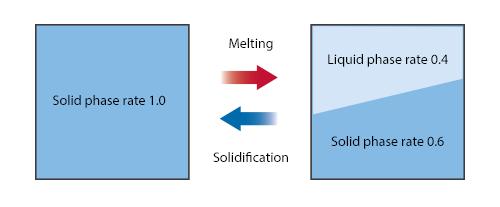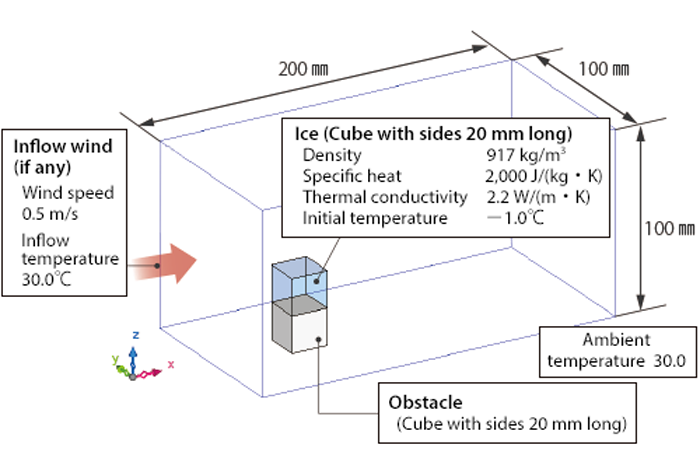# Master Course for Fluid Simulation Analysis of Multi-phase Flows by Oka-san: 19. Solidification/melting analysis III

#### Solidification/melting analysis III

This section continues to explain solidification/melting analysis. Until the previous section, the analysis target was a flow in which the liquid phase and the solid phase of the same substance were contained, like ice in water. In this final section of solidification/melting analysis, a gas-liquid-solid three-phase flow is discussed, including a gas phase in the case that ice in air melts into water.

When a solid melts into a liquid, the volume fraction of the solid (solid phase rate) is converted into the volume fraction of the liquid (liquid phase rate). Like the volume of water that changes as water freezes into ice, the volumes of a liquid and solid are not the same in many cases. Since it is difficult to consider this volume change in fluid simulation analyses, it is assumed here that the volumes of the liquid and solid are the same as shown in Figure 1.Figure 1　Relationship between solid phase rate and liquid phase rate

With elements in which a liquid and a solid coexist, the flow is solved only with elements whose solid phase rate is equal to or less than the liquid limit solid phase rate and other elements are assumed to be static. This modeling was already discussed last time. The flow of the liquid phase generated by melting is analyzed by using the free surface flow analysis method. Here is an example using the MARS (Multi-interface Advection and Reconstruction Solver) method introduced in this column previously.

A lot of you must have seen ice cream melting away immediately when they ate it near an electric fan. Here is a comparison of how ice melts when left in a room and when exposed to wind of room temperature. This time, this analysis is conducted by Atsushi Ueyama in our Engineering Department.

The analysis model is shown in Figure 2. Transient analysis is conducted until 300 seconds later with the latent heat of ice of 333,623 J/kg and the liquid limit solid phase rate of 0.7.Figure 2　Analysis model

The analysis result is shown in Figure 3. This animation shows an isosurface of a solid phase rate of 0.5 and a liquid phase rate of 0.5. You can see that (b) exposed to wind melts very quickly compared with (a) without wind. This is because the higher the wind speed is, the larger the coefficient of heat transfer becomes and the temperature of ice increases more rapidly.

Figure 3 (a): Isosurface of solid phase rate and liquid phase rate (no wind)

Figure 3 (b): Isosurface of solid phase rate and liquid phase rate (with wind)

Finally, calculation in case of ice cream is made in the same way.

Figure 4: Melting ice cream

The viscosity coefficient of the ice cream is assumed to be 10 times as high as that of water, and other material properties are the same as those of water and ice. Is the result convincing?

The times in animations in Figures 3 to 4 are accelerated by 10 times.

Solidification/melting analysis is concluded with this section. As explained before, not only analyses of solidification of liquid and melting of solid but also gas-liquid-solid three-phase flow analyses including gas phase have come to be possible. They can be applied to various targets of calculation.

The next column will explain "cavitation flow analysis."